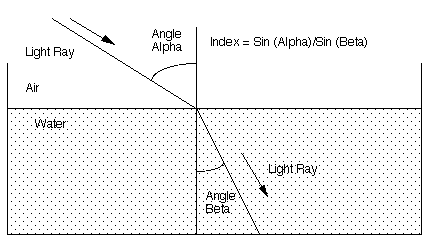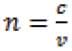# Snell's lawThis article was checked by pedagogue, but later was changed.
Article to be checked

```                           Snell's Law
```

Snell's law (or law of refraction) describes the change of directions of the propagation of light with straight-line character at the boundary between two media. This law is named after the Dutch astronomer and mathematician Willebrord van Roijen Snell and it is valid not only for optical phenomena but also for any kind of waves.

When light passes from one medium to another, one can often observe refraction as a consequence. The refraction is caused by the change of velocity of light waves. The velocity of light, which is a transverse electromagnetic wave, depends not on its wavelength or frequency but rather of the medium in which the light travels. The velocity of light decreases with increasing atom density of the medium. That means that the light is faster in air than in water.

With the formula of Snell's law one can calculate how much the light refracts when the light crosses the boundary: In order to measure the refractive ability of any given boundary, one compares the angle of refraction to the angle of incidence. The difference between both angles increases with increasing refraction. Both angles are measured with respect to the normal of the boundary. It is observed that the angle of incidence is bigger, when the velocity of the ray diminishes at the boundary. Due to this observation it holds:

picture:In this example the ray bends toward the normal like it does when it passes from air to water.

Picture:Usually it is more common to use the refracted index of the individual medium for this equation. The refracted index (n) of a medium is defined as the ratio of the speed of light in vacuum (c), which has a value of 3 x 108 m/s, to the speed (v) in the medium: �

picture:The lower the velocity is in a specific medium, the higher is the refracted index. In rewritten form, which is the actual form of Snell's law, the equation is called:

picture:��

Sources:

František Vítek, Lectures on Biophyics with medical orientation http://www.physicsclassroom.com/class/refrn/u14l2b.cfm http://www.math.ubc.ca/~cass/courses/m309- 01a/chu/Fundamentals/snell.htm http://de.wikipedia.org/wiki/Snelliussches_Brechungsgesetz